Courses

# Test: Reaction Of Alcohols- II

## 23 Questions MCQ Test Chemistry for JEE | Test: Reaction Of Alcohols- II

Description
This mock test of Test: Reaction Of Alcohols- II for JEE helps you for every JEE entrance exam. This contains 23 Multiple Choice Questions for JEE Test: Reaction Of Alcohols- II (mcq) to study with solutions a complete question bank. The solved questions answers in this Test: Reaction Of Alcohols- II quiz give you a good mix of easy questions and tough questions. JEE students definitely take this Test: Reaction Of Alcohols- II exercise for a better result in the exam. You can find other Test: Reaction Of Alcohols- II extra questions, long questions & short questions for JEE on EduRev as well by searching above.
QUESTION: 1

### Only One Option Correct Type Direction (O. Nos. 1-8) This section contains 8 multiple choice questions. Each question has four choices (a), (b), (c) and (d), out of which ONLY ONE is correct. Q.  Arrange the following alcohols in the increasing order of reactivity with HBr. I. benzyl alcohol II. p-methyl benzyl alcohol III. p-nitrobenzyl alcohol IV. p-chlorobenzyl alcohol

Solution:

Reactive intermediate carbocation is involved, hence greater the stability of carbocation, greater the reactivity of corresponding alcohols. Methyl group gives electron donating + I-effect, increases stability of carbocation, nitro group and chloro have both - I-effect, decreases stability of carbocation. Nitro group has stronger electron withdrawing power than chloro, nitro derivative is further less reactive than chloro derivative.

QUESTION: 2

### Which is the most appropriate reagent for the following oxidation reaction? CH3 —CH = CH — CH2OH → CH3 —CH =CH — COOH

Solution:

It is Jone's reagent which does not affect the olefinic bonds.

QUESTION: 3

### What is the major product of the following reaction ?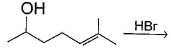Solution: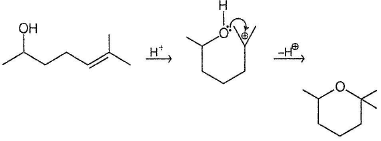QUESTION: 4

Which of the following is true statem ent regarding reaction of c/s and trans 2-hexene with CH3OH/H+ ?

Solution:

Both cis and trans 2-hexene form s the same carbocation, hence react at same rate.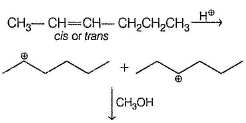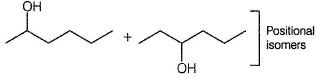QUESTION: 5

The major product formed in the following reaction is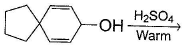Solution: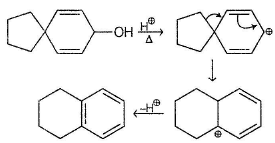QUESTION: 6

The relative rate of reaction of the following with H2SO4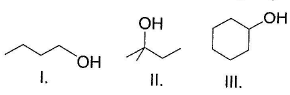Solution:

Reaction proceeds via carbocation intermediates. Hence, greater the stability of carbocation, greater the reactivity.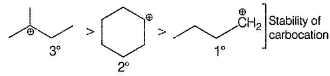QUESTION: 7

The major product in the following reaction is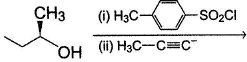Solution: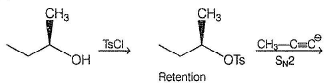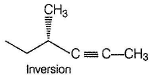QUESTION: 8

What is the major product of the following reaction?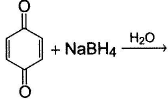Solution: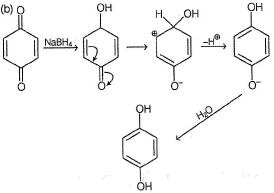*Multiple options can be correct
QUESTION: 9

One or More than One Options Correct Type

Direction (0 . Nos. 9-14) This section contains 6 multiple choice questions. Each question has four choices (a), (b), (c) and (d), out of which ONE or MORE THAN ONE are correct.

Q.

What is/are the expected product(s) in the following reaction?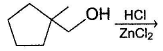Solution:

Reaction proceeds via carbocation intermediates.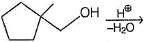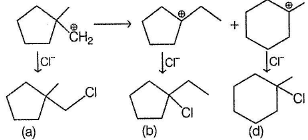*Multiple options can be correct
QUESTION: 10

What is/are the possible dehydration product(s) in the following reaction?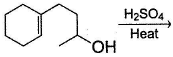Solution: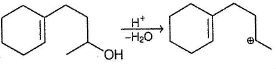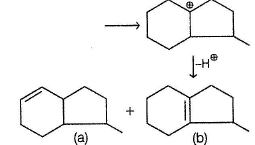*Multiple options can be correct
QUESTION: 11

What is/are the possible dehydration product(s) in the following reaction?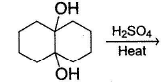Solution: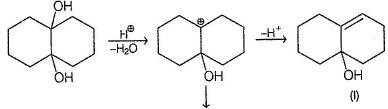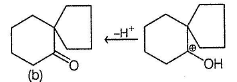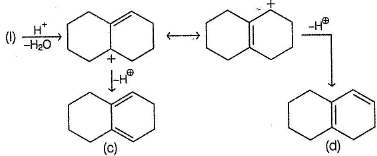*Multiple options can be correct
QUESTION: 12

In which of the following reaction(s), reactant and product(s) are correctly matched ?

Solution: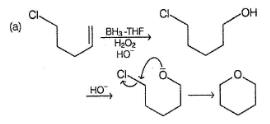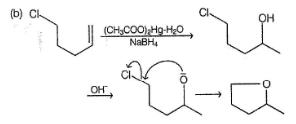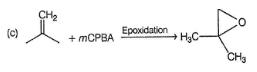(d) No SN2 reaction on C6H5Br.

*Multiple options can be correct
QUESTION: 13

In the following dehydration reaction, expected product(s) is/are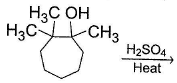Solution: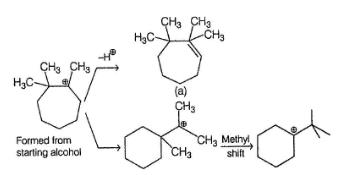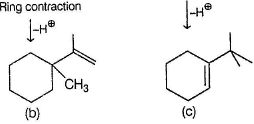*Multiple options can be correct
QUESTION: 14

Upon treatment with bromine water, allyl bromide gives chiefly primary alcohol BrCH2CHBrCH2OH. What are the expected primary alcohols in the following reaction ?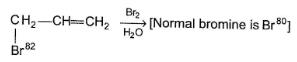Solution: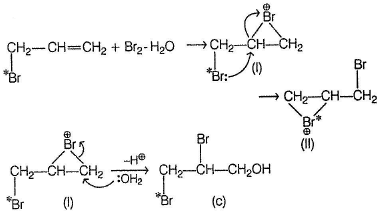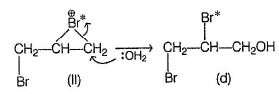Nucleophilic attack of H2O occur at α-C of cyclic bromonium ion where positive charge is more easily accommodated.

QUESTION: 15

Comprehension Type

Direction (Q. Nos. 15-19) This section contains 2 paragraphs, each describing theory, experiments, data, etc. Five questions related to the paragraphs have been given. Each question has only one correct answer among the four given options (a), (b), (c) and (d).

Passage I

An unknown organic compound A is determined to have molecular formula C6H12O and passing it through chiral column does not separate it into enantiomers. A does not react with Br2 nor with cold, dilute alkaline KMnO4. Heating A with concentrated H2SO4 gives product B (C6H10) which can be separated into enantiomers. Ozonolysis of a single enantiomer of B produces C, an optically active keto-aldehyde of formula C6H10O2 which gives positive iodoform test.

Q.

Structure of B that satisfy the above criteria is

Solution: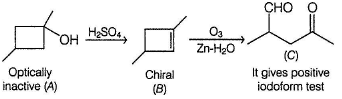QUESTION: 16

Passage I

An unknown organic compound A is determined to have molecular formula C6H12O and passing it through chiral column does not separate it into enantiomers. A does not react with Br2 nor with cold, dilute alkaline KMnO4. Heating A with concentrated H2SO4 gives product B (C6H10) which can be separated into enantiomers. Ozonolysis of a single enantiomer of B produces C, an optically active keto-aldehyde of formula C6H10O2 which gives positive iodoform test.

Q.

The correct statement regarding C is

Solution: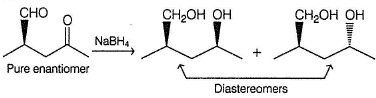QUESTION: 17

Passage I

An unknown organic compound A is determined to have molecular formula C6H12O and passing it through chiral column does not separate it into enantiomers. A does not react with Br2 nor with cold, dilute alkaline KMnO4. Heating A with concentrated H2SO4 gives product B (C6H10) which can be separated into enantiomers. Ozonolysis of a single enantiomer of B produces C, an optically active keto-aldehyde of formula C6H10O2 which gives positive iodoform test.

Q.

The correct statement regarding the outcome of the following reaction is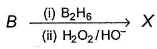Solution: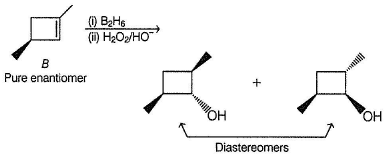Hydroboration oxidation brings about syn, anti-Markownikoff’s addition of water at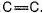QUESTION: 18

Passage II

An organic compound P (C10H18O)w hen treated with Br2(l) gives Q (C10H18OBr4). P on vigorous oxidation gives the following compounds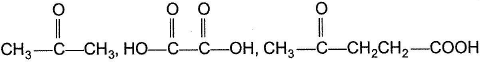Q.

What is the structural formula of P?

Solution: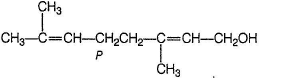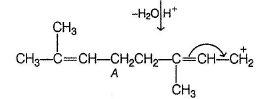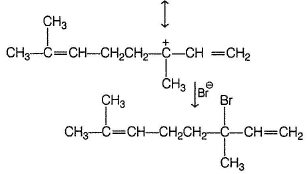X also gives the same bromide as it also involves the same carbocation in the final step.

QUESTION: 19

Passage II

An organic compound P (C10H18O)w hen treated with Br2(l) gives Q (C10H18OBr4). P on vigorous oxidation gives the following compoundsQ.

P on treatment with dilute acid undergo isomerisation to a more stable isomer R. What is the most likely structure of R ?

Solution: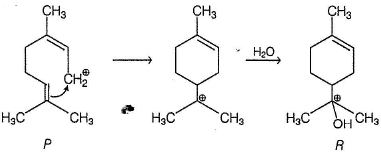*Answer can only contain numeric values
QUESTION: 20

One Integer Value Correct Type

Direction (Q. Nos. 20-23) This section contains 4 questions. When worked out will result in an integer from 0 to 9 (both inclusive).

In the reaction given below isotopic exchange occur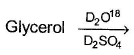Q.

What is the maximum gain in molecular mass observed?

Solution: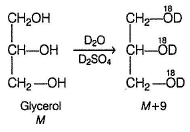via protonation, all —OH will beco me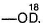, giving an increase in 3 units of mass per —OH group

*Answer can only contain numeric values
QUESTION: 21

Consider the following dehydration reaction,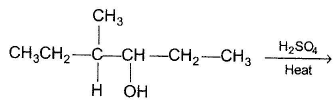Q.

If a pure enantiomer of alcohol is taken, then how many different alkenes are formed?

Solution: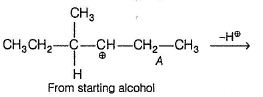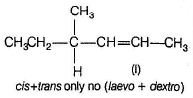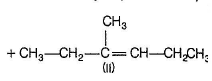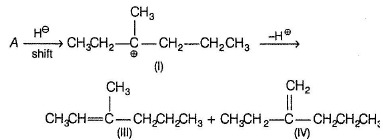Since, only a pure enantiomer A is taken, only c/s and frans-isomers of (I) would be produced. (II) will also be formed in cis-trans pair. (Ill) will also be formed as a pair of cis-trans isomer and (IV) will be a single isomer.

*Answer can only contain numeric values
QUESTION: 22

In the following reaction how many different diols, are formed?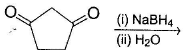Solution: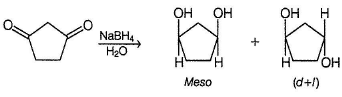*Answer can only contain numeric values
QUESTION: 23

If all carbonyls isomers of molecular mass = 86 u are separately treated with CH3MgBr followed by acid hydrolysis, how many of them will give racemic mixture ?

Solution:

The carbonyl C5H10O (CnH2nO = 86) has following isomers.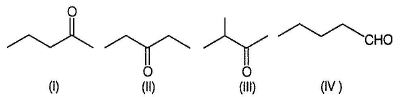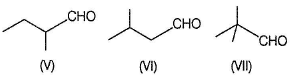Compounds (III), (IV), (VI) and (VII) gives racemic mixture with CH3MgBr. (I) and (II) gives achiral alcohols with CH3MgBr. (V) is enantiomeric, a pure enantiomer it gives pair of diastereomers with CH3MgBr.